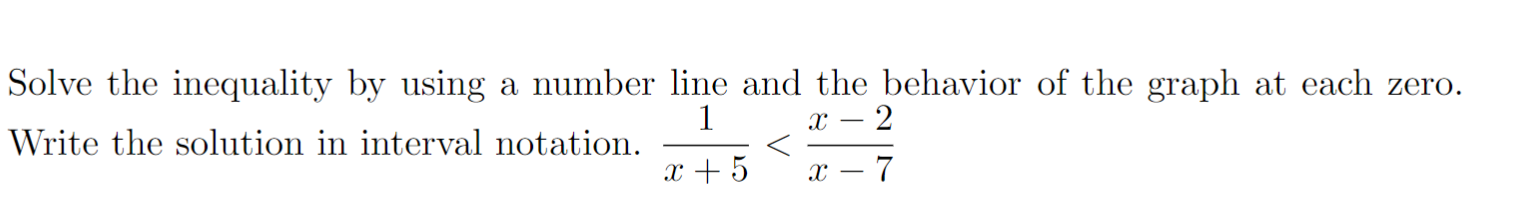# Solve the inequality by using a number line and the behavior of the graph at each zero.1c2Write the solution in interval notation5

Question
1 views

help!help_outlineImage TranscriptioncloseSolve the inequality by using a number line and the behavior of the graph at each zero. 1 c2 Write the solution in interval notation 5 fullscreen
check_circle

Step 1

Subtract (x-2)/(x-7) then subtract using LCD.

Step 2

Factor the numerator.

Step 3

We set every factor of the numerator and denominator eq...

### Want to see the full answer?

See Solution

#### Want to see this answer and more?

Solutions are written by subject experts who are available 24/7. Questions are typically answered within 1 hour.*

See Solution
*Response times may vary by subject and question.
Tagged in

### Other